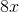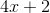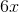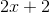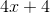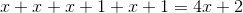ISEE Lower Level Math : How to find the perimeter of a rectangle

Example Questions

Example Question #21 : How To Find The Perimeter Of A Rectangle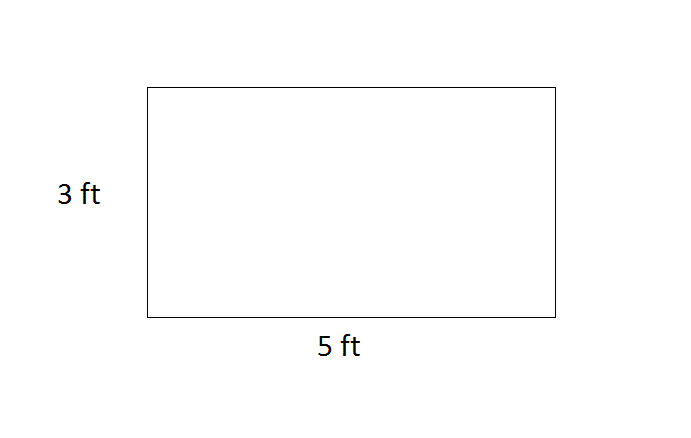What is the perimeter of the above rectangle?ftftftftftft

Explanation:

The perimeter of a rectangle is the length of all the sides added together. This rectangle has two short sides that are eachfeet long and two long sides that are eachfeet long.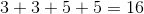, so the correct answer is.

Example Question #1051 : Isee Lower Level (Grades 5 6) Mathematics Achievement

Find the perimeter of a rectangle whose width isand length is.Explanation:

To solve, simply use the formula for the eprimeter of a rectangle. Thus,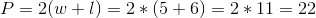Example Question #1052 : Isee Lower Level (Grades 5 6) Mathematics Achievement

Find the perimeter of a rectangle whose length isand width is.Explanation:

To solve, simply use the formula for the perimeter of a rectangle. Thus,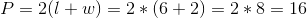Example Question #1053 : Isee Lower Level (Grades 5 6) Mathematics Achievement

You need to cover your rectangular driveway in blacktop but you need the area to figure out how much material to get.  You would just add up all four sides to get our answer.  Is this statement true or false?

False

True

False

Explanation:

The area of a rectangle is the length times the width not the sides added togther.  That would be the perimeter.

Example Question #1054 : Isee Lower Level (Grades 5 6) Mathematics Achievement

A computer screen has a height of 9 inches and a width of 16 inches. Find its perimeter. All answers are in inches.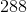Explanation:

A computer screen has a height of 9 inches and a width of 16 inches. Find its perimeter.

Perimeter is the distance around the outside of a shape. In this case, we are dealing with a rectangle. To find the perimeter of a rectangle, we need to add up all four sides, or use the following formula: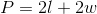Substitute our length and width into the equation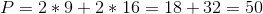So our perimeter is 50 inches

Example Question #1055 : Isee Lower Level (Grades 5 6) Mathematics Achievement

Your front yard is in the shape of a rectangle.  You want to build a fence all around your front yard.  The length of your yard is 40 feet.  The width of your yard is half the length.  How many feet of fencing will you need for your front yard?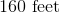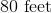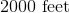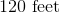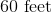Explanation:

To find how much fencing we will need, we need to determine the perimeter of the yard.  To find the perimeter, we use the following formula: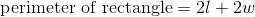where l is the length and w is the width of the rectangle (in this case, the yard).  We know the length is 40 feet.  We also know the width is half the length, so the width is 20 feet.  Now, we can substitute.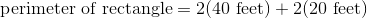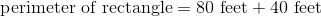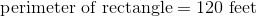Example Question #1056 : Isee Lower Level (Grades 5 6) Mathematics Achievement

A rectangle measuresinches on its short side, and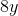inches on its long side.  What is its area?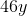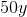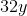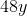Explanation:

To find the area of a rectangle, multiply the length of the long side by the length of the short side.  The best way to find the answer is: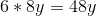Example Question #1057 : Isee Lower Level (Grades 5 6) Mathematics Achievement

A rectangle measures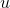inches on its short side, and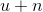inches on its long side.  What is its area?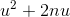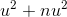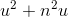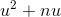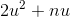Explanation:

To find the area of a rectangle, multiply the length of the long side by the length of the short side.  The best way to find the answer is: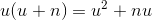Example Question #1058 : Isee Lower Level (Grades 5 6) Mathematics Achievement

A rectangle measuresinches along its short side, andinches along its long side.  What is the perimeter of the rectangle?Explanation:

To find the perimeter of any object, simply add the length of each side together.  The best answer is: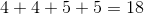Example Question #1059 : Isee Lower Level (Grades 5 6) Mathematics Achievement

A rectangle measuresinches along its short side, and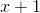inches along its long side.  What is the perimeter of the rectangle?